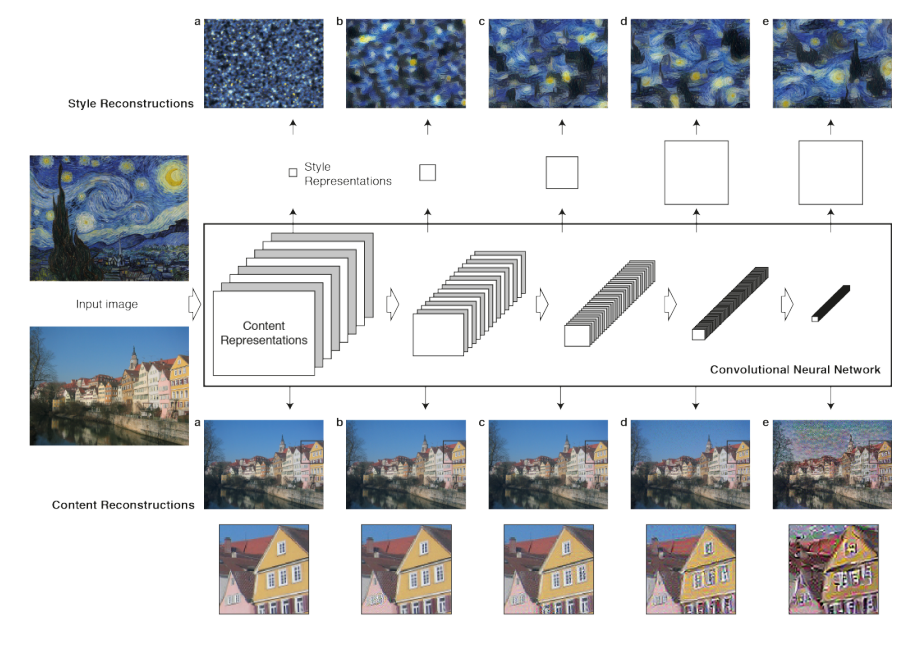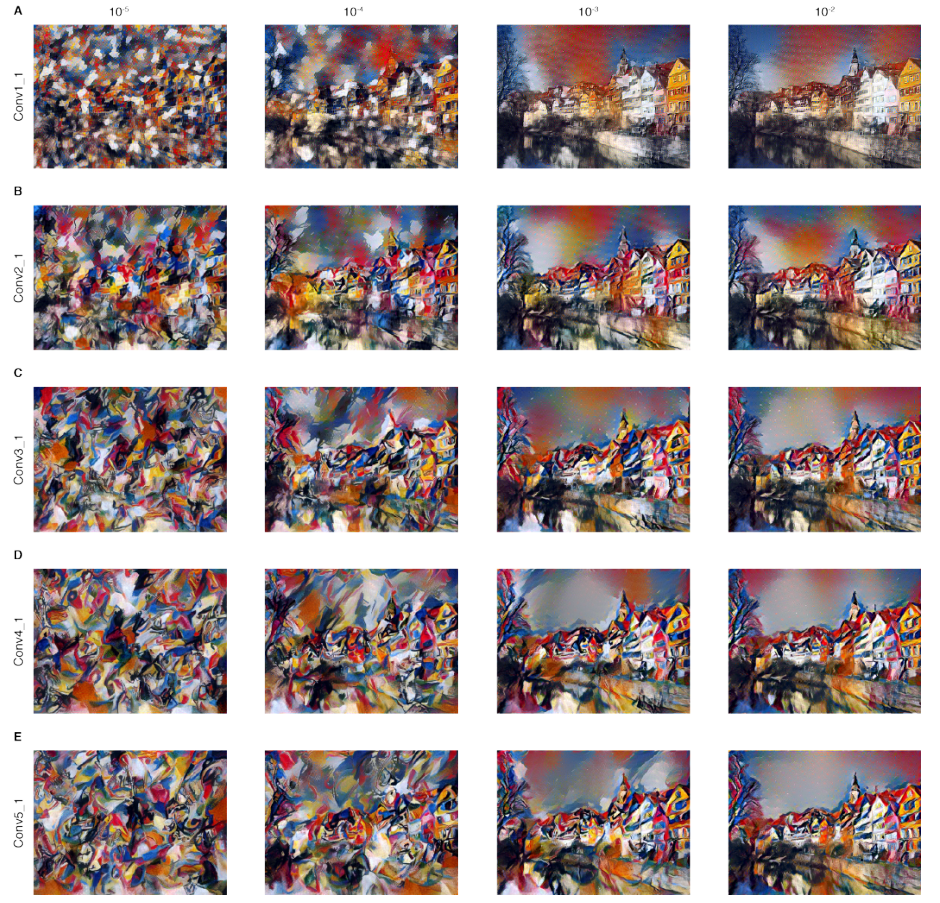# Neural Style Transform

Neural Style transfer is the technique of recomposing images in the style of other images using Deep Learning techniques and it has gotten very popular recently. I will be reading two papers related to Neural Style Transfer

CS231N: Convolutional Neural Networks for Visual Recognition. Starting with the first one, A Neural Algorithm of Artistic Style by Gatys, Ecker and Bethge.

• The paper introduces a system based on Deep Neural Networks that composes a interplay between the content and style of an image.

• The key finding of the paper is that representations of content and style in Convolutional Neural Networks (CNN) are separable. The system uses neural representations to separate and recombine the content style of images

### Content Representation

• Each layer of CNN can be understood as a collectin of image filters which outputs a differently filtered versins of the imput image
• When CNN are trained on object recognition, they develop a representation of the image that increasingly care about the actual content of the image, as opposed to its detailed pixel values
• Content Reconstruction: The inforamtion each layer contains about the input image can be visualized by reconstructing the image only from the feature map in that layer

### Style Representation

• To obtain a representation of the style of an input image, we use a feature space originally designed to capture texture information
• The feature space is built on top of the filter responses in each layer of the network and consists of the correlations between the different filters over the spatial extent of the feature maps. The style features captures the general appearance of the image in terms of color and local structures, which increases along the hierarchy
• Style Reconstruction: The information captured by style feature spaces built on different layers can be visualized by constructing an image that matches the style represntation##### Figure 1:
• Top half shows that reconstructing style feature spaces with higher layers matches the style of a given image on an increasing scale while discarding information of the global arrangement of the scene.
• Bottom half shows that reconstructing ccontent with higher layers preserve high-level content of the image while losing detailed pixel information

### Style Transfer

• We can generate images that mix the content and style representation from two different source images
• While the global arrangement of the original photograph is preserved, the colors and local structures are provided by the artwork
• Style can be more local if only lower layers are included. Matching the style representations up to higher layers leads to larger local image structures, thus a smoother and more continuous visual experience##### Figure 2:
• Comparisions between rows shows that style representation is more global (smoother) when higher layers are used and more local (pixelated) when only lower layers are used
• Comparisions between columns shows different relative weightings between the content and style reconstruction

### Method

• The style transfer result were generated on the basis of 19 layer VGG-Network, a CNN with 16 convolutional and 5 pooling layers.
• The fully connected layers are not used.
• For image synthesis, max-pooling was replaced by average pooling.
• A layer with $N_l$ distinct filters has $N_l$ feature maps each of size $M_t$, where $M_t$ is the height times the width of the feature map. The response in a layer $l$ can be stored in a matrix $F^l \in \mathcal{R}^{N_t \times M_t}$

#### Content Reconstruction

• To visualize the image information encoded at different layers of the hierarchy, gradient descent is performed on a white noise image to find another image $\overset{\rightarrow}{x}$ with feature responses $F^l$ that matches the feature responses $P^l$of the original image $\overset{\rightarrow}{p}$. We define the loss as the L-2 loss between the feature representations $$\mathcal{L}_{content}(\overset{\rightarrow}{p}, \overset{\rightarrow}{x}, P^l, F^l) = \frac{1}{2} \sum_{i,j} (F_{ij}^l - P_{ij}^l)^2$$

• The derivative of the loss is $$\frac{\partial \mathcal{L}}{\partial F_{ij}^l} = \begin{cases} (F_{ij}^l - P_{ij}^l), & F_{ij}^l > 0 \\ 0, & F_{ij}^l < 0 \end{cases}$$

#### Style Reconstruction

• The style representation is built by computing the correlations between the different filter responses. The feature correlations are given by the Gram matrix $G^l \in \mathcal{R}^{N_t \times N_l}$, where $G^l_{ij}$ is the inner product between the feature map $i$ and $j$ in layer $l$ $$G^l_{ij} = \sum_k F^l_{ik}F^l_{jk}$$
• To generate a texture that matches the style of a given image, gradient descent is performed on a white noise image to find another image $\overset{\rightarrow}{x}$ with style representation $G^l$ that matches the style representation $A^l$of the original image $\overset{\rightarrow}{a}$. The contribution of each layer $l$ to the total loss is $$E_l = \frac{1}{4N_l^2M_l^2} \sum_{i,j} (G_{ij}^l - A_{ij}^l)^2$$ And the total loss is the weighted sum of the loss from each layer $l$ $$\mathcal{L}_{style} = \sum_l w_l E_l$$

• The derivative of $E_l$ with trespect to the activations in layer $l$ is $$\frac{\partial E_l}{\partial F_{ij}^l} = \begin{cases} \frac{1}{N_l^2M_l^2}[(F^l)^T(F^l - A^l)]_{ji}, & F_{ij}^l > 0 \\ 0, & F_{ij}^l < 0 \end{cases}$$

#### Style Transfer Reconstruction

• To mix the content of an image with the style of another image, we minimize the distance of a white noise image $\overset{\rightarrow}{x}$ from the content representation of the content image $\overset{\rightarrow}{p}$ in one layer of the network and the style representation of the style image $\overset{\rightarrow}{a}$ in a number of layers of the CNN

$$\mathcal{L}_{total}(\overset{\rightarrow}{p}, \overset{\rightarrow}{a}, \overset{\rightarrow}{x}) = \alpha \mathcal{L}_{content}(\overset{\rightarrow}{p}, \overset{\rightarrow}{x}) + \beta \mathcal{L}_{style}(\overset{\rightarrow}{a}, \overset{\rightarrow}{x})$$